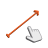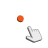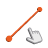Back to Adaptive Vision Library website

You are here: Start » Function Reference » Geometry 2D Interpolations

# Geometry 2D Interpolations

Select a function from the list below.

Icon Name Description LibraryAveragePoint Computes the middle point of two input points. LiteLerpAngles Linearly interpolates between two angles in the direction of minimum turn. LiteLerpPaths Linearly interpolates between two paths. LiteLerpPoints Linearly interpolates between two points. LiteLerpSegments Linearly interpolates between two segments. LiteLerpVectors Linearly interpolates between two vectors. LiteLerpVectors_Radial Linearly interpolates between two radial vectors. LiteMedianAngle Computes the median angle of the input angles. Lite